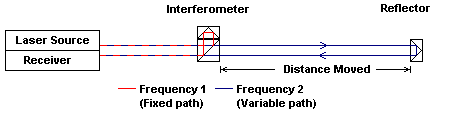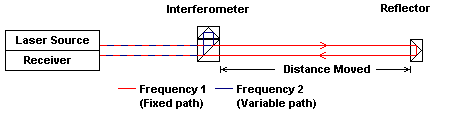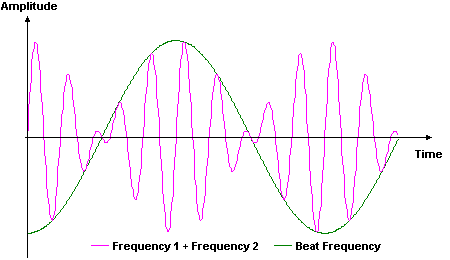Specialists in Engineering Metrology

 How do laser interferometers work ?

Interferometers use the interference of two light beams to perform relative measurements of displacement. The Agilent Laser Interferometer uses two known frequencies of laser light to take measurements. The two frequencies of light are split such that one of the frequencies follows a fixed path while the second frequency travels over the measurement path (figures 1 & 2).Figure 1. Path of Laser light in a basic interferometer

Stationary Interferometer Moving ReflectorFigure 2. Path of Laser light in a basic interferometer

Moving Interferometer Stationary Reflector

When the two frequencies are recombined, a ‘beat frequency’ is obtained (figures 3 & 4). As the path length of the second frequency is changed the beat frequency changes. By measuring the beat frequency the distance moved can be calculated.Figure 3. Two frequencies of lightFigure 4.  Interference of Frequencies 1 and 2 and the Beat Frequency

The simpler single frequency interferometer works in a similar manner to that of the two frequency system. Instead of two frequencies, two beams of light of the same frequency are split such that one of the light beams follows a fixed path while the second beam travels over the measurement path. When these two light beams interfere the intensity varies as the moving element causes the light path length to change. This method of measurement is much more stable than single frequency systems when air conditions are not perfect, such as can be expected when working on-site.